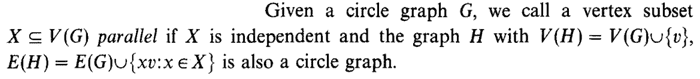# What does it mean? (Graph theory)

ff4ever
Hi,

In the paper "A triangle-free circle graph with chromatic number 5" from A.A. Ageev, I don't understand what the author mean with the following definition:Somebody can explain it to me? (I'm not mathematician).
Thanks !

http://www.sciencedirect.com/science/article/pii/0012365X95003492

Homework Helper
Gold Member
A graph is a set of spots ('vertices'), some of which are connected to each other by lines ('edges'). The arrangement of the spots and the shape of the connecting lines doesn't matter. All that matters is what spots are connected to each other.

A 'circle graph' is a particular type of graph whose meaning is explained here.

A set of vertices is 'independent' in a graph if, for every pair of vertices in the set, the graph has no edge connecting the two.

For a graph ##H##, we use ##V(H)## to refer to the set of vertices in the graph, and ##E(H)## to refer to the set of edges.

If ##x## and ##v## are vertices in a graph then ##xv## refers to an edge connecting ##x## to ##v##.

What the definition says is that a subset ##X## of vertices of a circle graph ##G## is defined to be parallel iff the graph formed from ##G## by adding a new vertex and adding edges joining the new vertex to every vertex in ##X## is also a circle graph.

ff4ever
Okay. Now I understand. Thanks a lot !